# Implement stack using a singly linked list

## Problem Statement

Implement stack using a singly linked list that supports the basic operations of stack data structure such as push(), pop(), top(), isEmpty(), and follows the last in first out order(LIFO).

### Basic operations of stack

• push(): it pushes an element into the stack data structure.
• pop(): it removes the top element of the stack.
• top_element(): it gives the value of the top element of the stack.
• isEmpty(): it returns true if the stack is empty else returns false.
• print_stack(): it prints the contents of the stack in LIFO order.

## Algorithm

To implement a stack using a singly linked list, we make some changes in the singly linked list to make it work as a stack. Here the top of the stack is the head of the linked list. Initially, the head pointer of the linked list is pointing to NULL.

### push()

We attach the new node in front of the linked list to follow LIFO(last in first out) order like the stack data structure.

Steps:

• Create a new node temp.
• Finally, make the temp node as the new head, head = temp.

Example:

• push(5)

• Create a temp node of the given value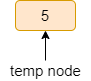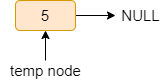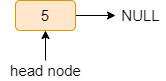• push(3)

Repeat the above steps.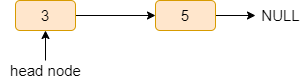### pop()

We remove the elements from the front of the linked list to follow the LIFO( last in first out) order as the stack data structure.

Steps:

• If the head is NULL, print “stack is empty”.
• Else create a temp node and store the head in the temp node.
• Delete the temp node.

Example:• pop()

Steps:

• Create a temp node and store the head of the list in it.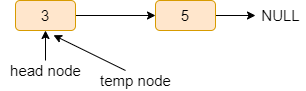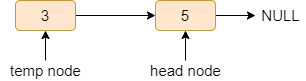• Delete temp.### top_element()

If the linked list is empty, print “stack is empty”. Else return the data of the head node.

### isEmpty()

If the head is pointing to the NULL, return true else, return false.

### print_stack()

Steps:

• Declare a curr pointer pointing to the head of the list.
• Run a while loop till curr!=NULL.
• Print the curr->data and do curr=curr->next.

You can also watch our video on this topic for a visual understanding of the agenda at hand.

### Code in C++

``````#include <bits/stdc++.h>
using namespace std;

struct node
{
int data;
node* next;
};

void push(int x)
{
node* temp;
temp = new node();
temp->data = x;
}

bool isEmpty()
{
return true;
else
return false;
}

int top_element()
{

{
cout << "stack is empty" << endl;
}
else

}

void pop()
{
node* temp;

if (isEmpty())
{
cout << "stack is empty" << endl;
}
else
{
delete(temp);
}
}

void print_stack()
{
struct node* curr;

if (isEmpty())
{
cout << "stack is empty" << endl;
}
else
{
cout << "Elements are: ";
while (curr != NULL)
{

cout << curr->data << " ";

curr = curr->next;
}
cout << endl;
}
}

int main()
{

push(5);
push(3);
push(6);
print_stack();

isEmpty();
cout << "Top: "
<< top_element() << endl;

pop();
print_stack();

cout << "Top: "
<< top_element() << endl;

return 0;
}``````

#### Output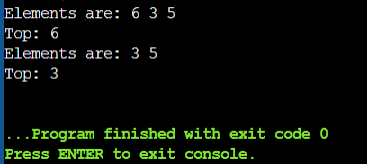### Code in Java

``````// Main class
public class Main {
static class StackNode {
int data;
StackNode next;
StackNode(int data) {
this.data = data;
}
}

StackNode root;

public void push(int data)
{
StackNode newNode = new StackNode(data);
if (root == null) {
root = newNode;
}
else {
StackNode temp = root;
root = newNode;
newNode.next = temp;
}
}

// pop method pop element from head and return it's data
public int pop()
{
int popped;
if (root == null) {
System.out.println("Stack is Empty");
return 0;
}
else {
popped = root.data;
root = root.next;
return popped;
}
}

// top_element will return the top of the stack
public int top_element()
{
if (root == null) {
System.out.println("Stack is empty");
return Integer.MIN_VALUE;
}
else {
return root.data;
}
}

public static void main(String[] args)
{
Main obj = new Main();
obj.push(5);
obj.push(2);
obj.push(8);
obj.push(7);

System.out.println(obj.top_element());
System.out.println(obj.pop());

System.out.println(obj.top_element());
System.out.println(obj.pop());

System.out.println(obj.top_element());
System.out.println(obj.pop());

}
}``````

#### Output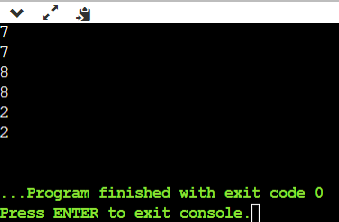### Code in Python

``````
# Node class
class Node:
def __init__(self,data):
self.data = data
self.next = None

# Stack class
class Stack:

# constructor initializing head with None
def __init__(self):

# Checks if stack is empty
def isempty(self):
return True if self.head == None else False

# push method adds element to the start of stack
def push(self,data):
else:
new_node = Node(data)

# returns the data of the head node
def topElement(self):
if self.isempty():
return None
else:

# pop method will remove the current head and return its value
def pop(self):
if self.isempty():
return None
else:
popped_node.next = None
return popped_node.data

# Driver code
stack_obj = Stack()

stack_obj.push(5)
stack_obj.push(2)
stack_obj.push(7)
stack_obj.push(8)

print(stack_obj.topElement())
print(stack_obj.pop())

print(stack_obj.topElement())
print(stack_obj.pop())

print(stack_obj.topElement())
print(stack_obj.pop())

``````

#### Output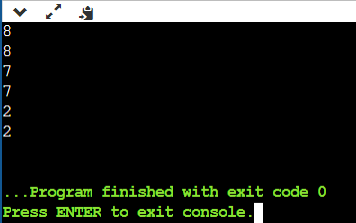### Complexity Analysis

Time complexity:

• push(): O(1), as we are not traversing the whole list.
• pop():  O(1), as we are not traversing the entire list.
• isEmpty(): O(1), as we are checking only the head node.
• top_element(): O(1), as we are printing the head node.
• print_stack(): As we are traversing through the whole list it will be O(n), where n is the number of nodes present in the linked list.

Space complexity: O(1), as we require constant extra space.

## FAQs

#### What is a stack?

A stack is a linear data structure in which operations are performed in a specific order. The order can be LIFO (last-in, first-out) or FILO (first in, first out) (First In Last Out).

#### What does the stack work?

A stack is a type of linear data structure in which elements are stacked on top of one another. Only the most recent element added, i.e. the element at the top of the stack, can be accessed. A stack, in other words, is a Last In First Out (LIFO) structure. It is the inverse of a queue, which operates on a first-come, first-served basis (FIFO).

## Conclusion

So, this article discussed the approach to implement stack using a singly linked list and all the steps to implement the basic operations of the stack like push(), pop(), top() and isEmpty() with examples and code in C++.

If you are a beginner, interested in coding and want to learn DSA, you can look for our guided path for DSA, which is free!